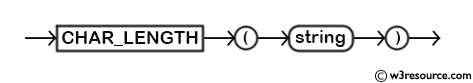# MySQL CHAR_LENGTH() function

## CHAR_LENGTH() function

MySQL CHAR_LENGTH() returns the length (how many characters are there) of a given string. The function simply counts the number characters and ignore whether the character(s) are single-byte or multi-byte. Therefore a string containing three 2-byte characters, LENGTH() function will return 6, whereas CHAR_LENGTH() function will returns 3.

Syntax:

`CHAR_LENGTH (string)`

Argument:

Name Description
string A string whose length is to be retrieved.

Syntax Diagram:MySQL Version: 5.6Video Presentation:

Example: MySQL CHAR_LENGTH() function

The following MySQL statement will count the length of the string specified as an argument.

Code:

``````SELECT CHAR_LENGTH('test string');
```
```

Sample Output:

```mysql> SELECT CHAR_LENGTH('test string');
+----------------------------+
| CHAR_LENGTH('test string') |
+----------------------------+
|                         11 |
+----------------------------+
1 row in set (0.00 sec)
```

Example of MySQL CHAR_LENGTH() function with where clause

The following MySQL statement will count how many characters are there in the names of publishers (pub_name) from the publisher table, and returns the name and number of characters in the names if the name has more than twenty characters.

Code:

``````SELECT pub_name,
CHAR_LENGTH(pub_name)  AS 'character length'
FROM publisher
WHERE CHAR_LENGTH(pub_name)>20;
```
```

Sample table: publisher

Sample Output:

```mysql> SELECT pub_name,
-> CHAR_LENGTH(pub_name) AS 'character length'
-> FROM publisher
-> WHERE CHAR_LENGTH(pub_name)>20;
+------------------------------+------------------+
| pub_name                     | character length |
+------------------------------+------------------+
| New Harrold Publication      |               23 |
| Summer Night Publication     |               24 |
| Pieterson Grp. of Publishers |               28 |
+------------------------------+------------------+
3 rows in set (0.00 sec)
```

PHP script:

``````<!doctype html>
<html lang="en">
<meta charset="utf-8">
<meta name="viewport" content="width=device-width, initial-scale=1">
<title>example-char_length-function - php mysql examples | w3resource</title>
<meta name="description" content="example-char_length-function - php mysql examples | w3resource">
<body>
<div class="container">
<div class="row">
<div class="col-md-12">
<h2>List of publisher's name and its character length, only if the character length is more than twenty:</h2>
<table class='table table-bordered'>
<tr>
<th>Publisher's Name</th><th>Character length</th>
</tr>
<?php
\$hostname="your_hostname";
\$db = "your_dbname";
foreach(\$dbh->query('SELECT pub_name,CHAR_LENGTH(pub_name)
AS "character length"
FROM publisher
WHERE CHAR_LENGTH(pub_name)>20') as \$row) {
echo "<tr>";
echo "<td>" . \$row['pub_name'] . "</td>";
echo "<td>" . \$row['character length'] . "</td>";
echo "</tr>";
}
?>
</tbody></table>
</div>
</div>
</div>
</body>
</html>
```
```
View the example in browser

JSP script:

``````%@page contentType="text/html" pageEncoding="UTF-8"%>
<%@ page import="java.sql.*" %>
<%@ page import="java.io.*" %>
<!DOCTYPE html>
<html>
<meta http-equiv="Content-Type" content="text/html; charset=UTF-8">
<title>example-char_length-function</title>
<body>
<%
try {
Class.forName("com.mysql.jdbc.Driver").newInstance();
String Host = "jdbc:mysql://localhost:3306/w3resour_bookinfo";
Connection connection = null;
Statement statement = null;
ResultSet rs = null;
connection = DriverManager.getConnection(Host, "root", "datasoft123");
statement = connection.createStatement();
String Data ="SELECT pub_name,CHAR_LENGTH(pub_name) AS 'character length' FROM publisher WHERE CHAR_LENGTH(pub_name)>20";
rs = statement.executeQuery(Data);
%>
<TABLE border="1">
<tr width="10" bgcolor="#9979">
<td>Publisher's Name</td>
<td>Character length</td>
</tr>
<%
while (rs.next()) {
%>
<TR>
<TD><%=rs.getString("pub_name")%></TD>
<TD><%=rs.getString("character length")%></TD>
</TR>
<%   }    %>
</table>
<%
rs.close();
statement.close();
connection.close();
} catch (Exception ex) {
out.println("Cant connect to database.");
}
%>
</body>
</html>
```
```

MySQL - LENGTH() vs CHAR_LENGTH()

We have already discussed in description section that LENGTH() function returns the length of the string calculated by number bytes whereas CHAR_LENGTH() function returns the length of the string calculated by numbers of characters. See the following example :

```mysql> select length('§'), char_length('§');
+------------+-----------------+
| length('§')| char_length('§')|
+------------+-----------------+
| 2          | 1               |
+------------+-----------------+
1 row in set (0.00 sec)
```

The section sign (§, Unicode U+00A7) occupies 2 bytes even though it is a single character.

Online Practice Editor:

All String FunctionsPrevious: BIT_LENGTH
Next: CHAR

﻿